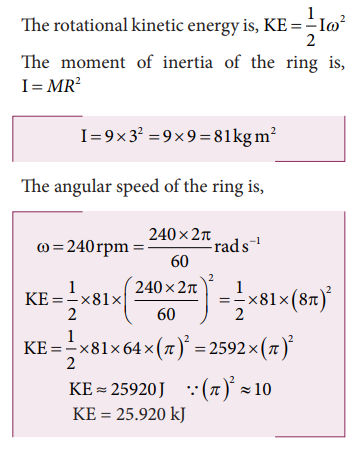Home | | Physics 11th std | Solved Example Problems for Kinetic Energy in Rotation

# Solved Example Problems for Kinetic Energy in Rotation

Physics : Motion of System of Particles and Rigid Bodies : Kinetic Energy in Rotation

Solved Example Problems for Kinetic Energy in Rotation

### Example 5.20

Find the rotational kinetic energy of a ring of mass 9 kg and radius 3 m rotating with 240 rpm about an axis passing through its center and perpendicular to its plane. (rpm is a unit of speen of rotation which means revolutions per minute)

### SolutionStudy Material, Lecturing Notes, Assignment, Reference, Wiki description explanation, brief detail
11th Physics : UNIT 5 : Motion of System of Particles and Rigid Bodies : Solved Example Problems for Kinetic Energy in Rotation |